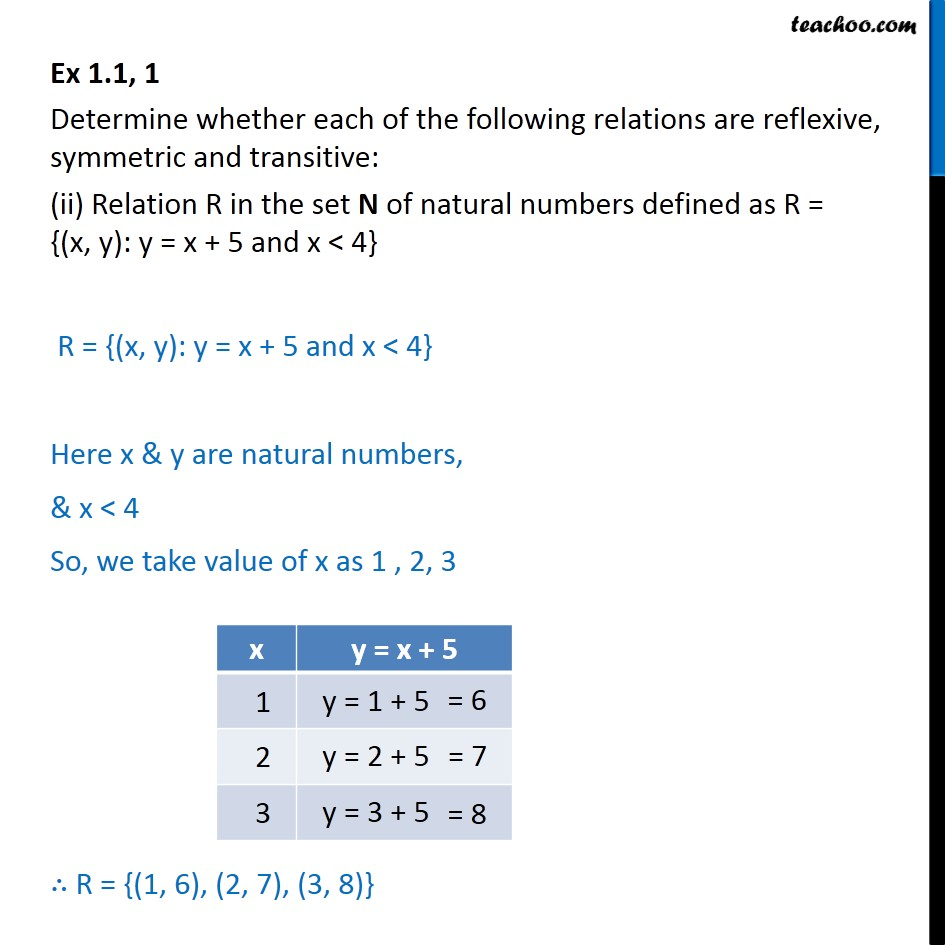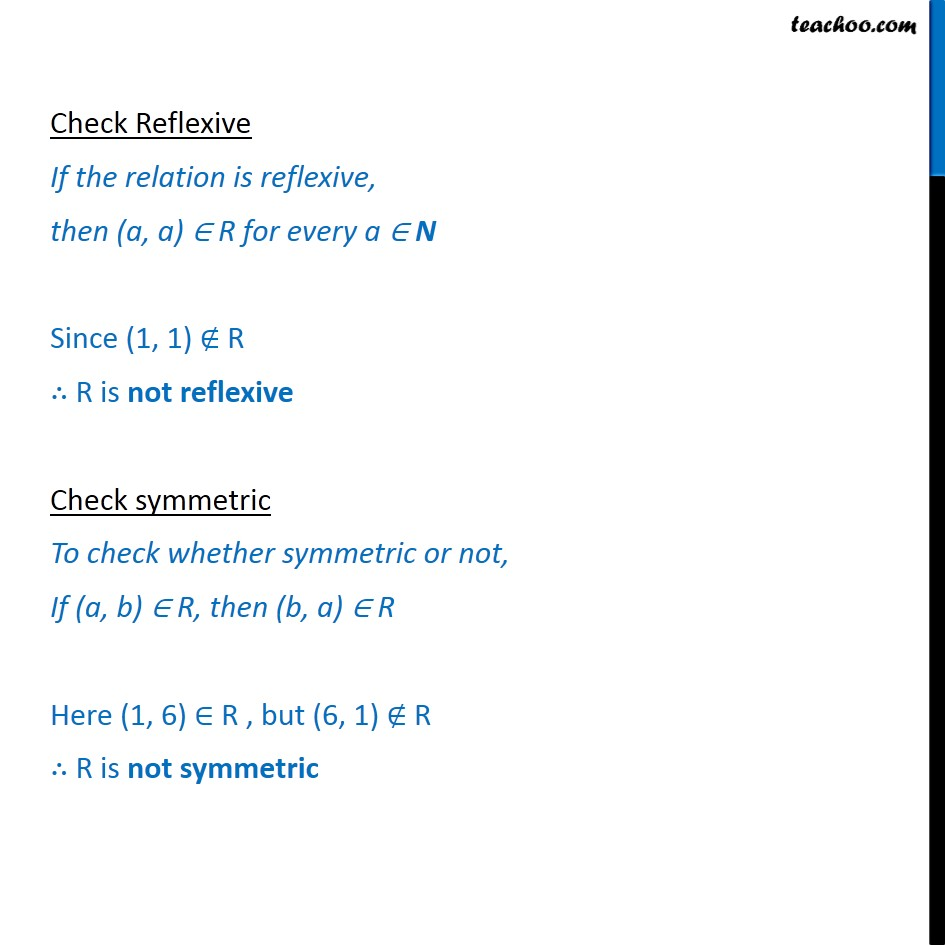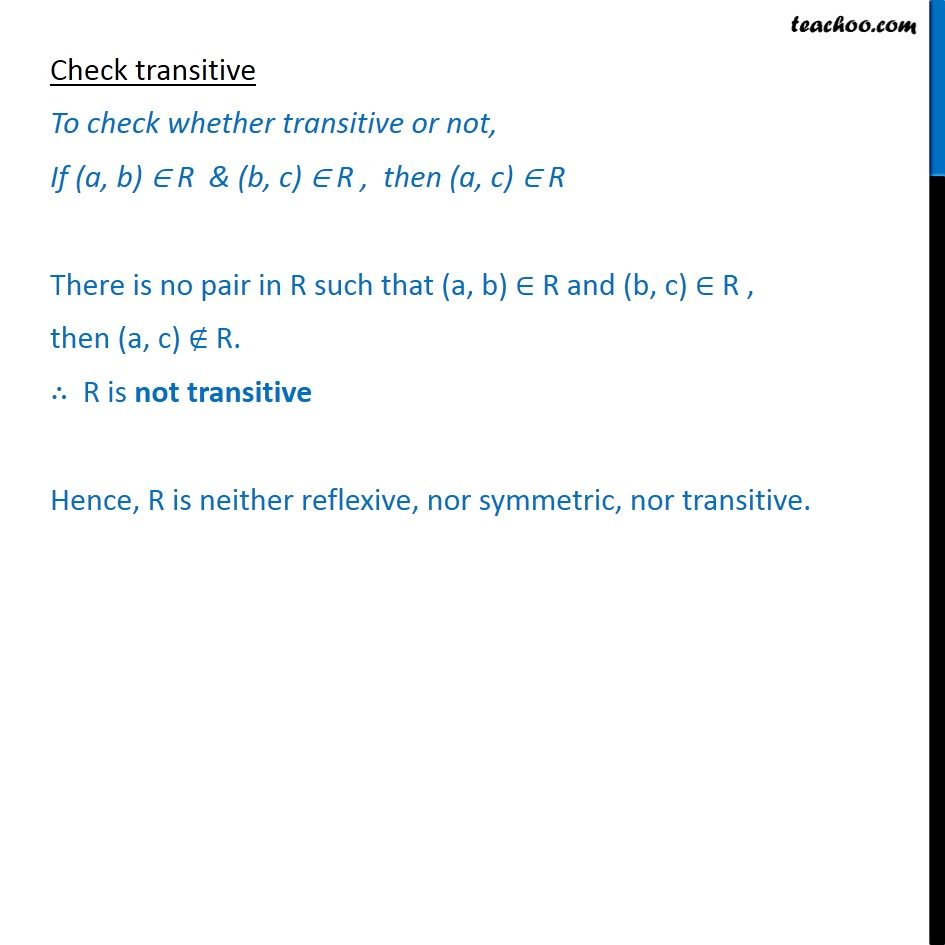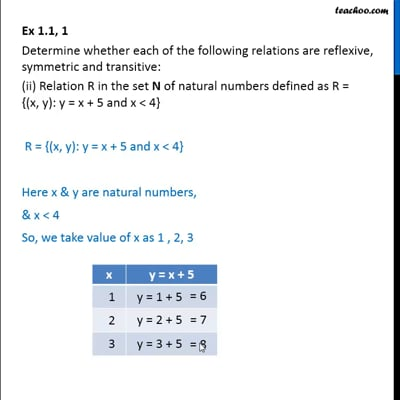Ex 1.1

Chapter 1 Class 12 Relation and Functions
Serial order wiseThis video is only available for Teachoo black users

Maths Crash Course - Live lectures + all videos + Real time Doubt solving!

### Transcript

Ex 1.1, 1 Determine whether each of the following relations are reflexive, symmetric and transitive: (ii) Relation R in the set N of natural numbers defined as R = {(x, y): y = x + 5 and x < 4} R = {(x, y): y = x + 5 and x < 4} Here x & y are natural numbers, & x < 4 So, we take value of x as 1 , 2, 3 R = {(1, 6), (2, 7), (3, 8)} Check Reflexive If the relation is reflexive, then (a, a) R for every a N Since (1, 1) R R is not reflexive Check symmetric To check whether symmetric or not, If (a, b) R, then (b, a) R Here (1, 6) R , but (6, 1) R R is not symmetric Check transitive To check whether transitive or not, If (a, b) R & (b, c) R , then (a, c) R There is no pair in R such that (a, b) R and (b, c) R , then (a, c) R. R is not transitive Hence, R is neither reflexive, nor symmetric, nor transitive.